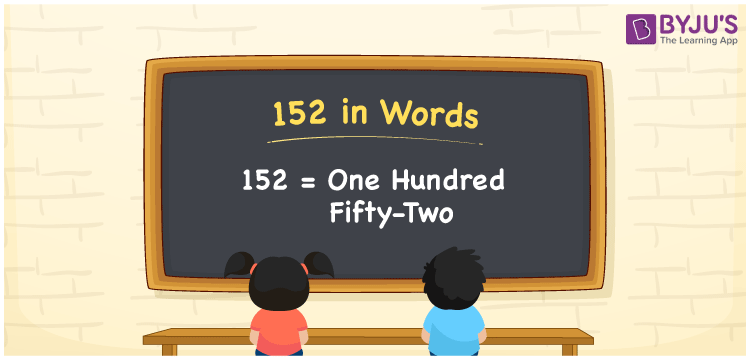# 152 in Words

152 in words is written as One Hundred Fifty-two. The name of number 152 in English is “One Hundred Fifty-two”. The word One Hundred Fifty-two is used in many real-life situations. For example, there are One Hundred Fifty-two people in a market. Thus, it is a counting number. Also, to represent the currency equal to 152, we can write it in words as Rupees One Hundred Fifty-two or One Hundred Fifty-two rupees. Therefore, it is necessary to learn the numbers in words, for the ease of understanding and expressing them.

 152 in words One Hundred Fifty-two One Hundred Fifty-two in Numbers 152

## 152 in English Words## How to Write 152 in Words?

If we know the place value of digits of 152, then we can easily express it in words. The place value is basically the position of a digit in a number. 152 is a three-digit number, therefore, we can specify the position of each digit of 152 in a place value chart. In the Indian numbering system, the order of place value of digits from right to left is given by:

 Hundreds Tens Ones 1 5 2

We can write it in expanded form as:

1 × Hundred + 5 × Ten + 2 × One

= 1 × 100 + 5 × 10 + 2 × 1

= 100 + 50 + 2

= 152

= One Hundred Fifty-two

Therefore, 152 in words is written as One Hundred Fifty-two.

152 is a natural number that precedes 153 and succeeds 151.

152 in words – One Hundred Fifty-two

Is 152 an odd number? – No

Is 152 an even number? – Yes

Is 152 a perfect square number? – No

Is 152 a perfect cube number? – No

Is 152 a prime number? – No

Is 152 a composite number? – Yes

## Frequently Asked Questions on 152 in Words

### How to write 152 in words?

152 in English is written as “One Hundred Fifty-two”.

### Is 152 an odd number or even number?

152 is an even number because it is wholly divisible by 2.

### Write One Hundred Fifty-two in numbers.

One Hundred Fifty-two in numbers is 152.